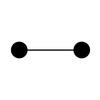# Using the loop construct, add the current value of number to the numbers array. Inside of the loop, add 1 to the number

What am i doing wrong?

loop.rb
```numbers = []

number = 0

loop do
number =+ 1
number.push(numbers)
if numbers == 3
break
end
end

Bummer! NoMethodError: undefined method `push' for 0:Fixnum Did you mean? puts
```MOD

Hi Philip,

You want to `push` onto the `numbers` array; pass in `number` as the method argument. Your error is saying that `number` doesn't have a `push` method - that's because it isn't an array; `numbers` is!```numbers.push(number)
```

Also, you want to do that first in the loop, before you increment `number` otherwise your array will never have the value zero in it. The question wants add the current value of number to the numbers array - this includes the initial zero value. Next up; to increment, use `+=` rather than `=+`.

That all looks like:

```loop do
numbers.push(number)
number += 1
break if number == 3
end
```

Let me know how you get on.

Steve.